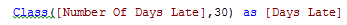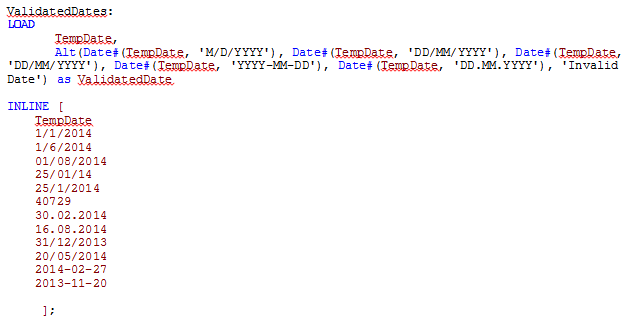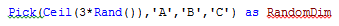# Design

The Design blog is all about product and Qlik solutions, such as scripting, data modeling, visual design, extensions, best practices, and more!

Announcements
QlikWorld 2023, a live, in-person thrill ride. Save \$300 before February 6: REGISTER NOW!Employee

## Which conditional functions do you use?

What does Class, Alt, Pick, If, Match, Mixmatch and Wildmatch all have in common?  They are all conditional functions that can be used in the QlikView script or in the user interface.  Personally, I have only used two of them – Class and If which is why I thought it would be interesting to learn a little more about them and their capabilities.  These functions return a value based on a comparison or a condition.  Let’s take a quick look at each of these.

Class

Class allows you to create buckets/groupings of data.  For example, assume you have a data model with order data and there is a field with the number of days an order is late.  Using class, the number of days can be grouped based on an interval we select.  The expression below will create buckets with 30 day intervals:The buckets will look like the image below.Alt

The Alt function will return the first parameter that has a valid number representation (including dates).  So in the script below, I can use Alt to check the format of the dates in the TempDate field.  If the format matches one of the parameters, then it is returned otherwise the last parameter in the Alt function is returned.  The order of the parameters indicates the priority order so that can be used to determine how the dates should be interpreted.  For example, should 7/4/2014 be interpreted as the 4th of July in the US or the 7th of April in the UK?Here are the results:The TempDate is the original date and the ValidatedDate is the value returned by the Alt function.

Pick

The Pick function will return the expression/value corresponding to the expression that matches the first parameter.  For example, Pick(2, ‘A’, ‘B’, ‘C”) will return B because B is the second expression and Pick(Number, Sum(1+1), Sum(2+2), Sum(3+3), Sum(4+4), Sum(5+5)) will return 6 if Number = 3.  This function is excellent when you want to generate a random field value, e.g.If

Everyone has used an If statement at some point whether in QlikView or some other programming language.

If(condition, then , else)

The If statement check a condition (the first parameter) and if the condition is true, it returns the “then” parameter otherwise it returns the “else” parameter.

For example, if(1+1=2, ‘Woo hoo, I can add’, ‘Need more practice’).

Match, Mixmatch & Wildmatch

These functions are very similar in that they all perform a comparison.

• The Match function does a case sensitive comparison between the first parameter and the expressions

match( str, expr1 [ , expr2,...exprN ] )

Match(X, ‘Jan’, ‘Feb’, ‘Mar’, ‘Apr’)

If X=Jan, 1 is returned

If X=’Feb’, 2 is returned

• The Mixmatch function does a case insensitive comparison between the first parameter and the expressions

mixmatch( str, expr1 [ , expr2,...exprN ] )

Mixmatch(X, ‘Jan’, ‘Feb’, ‘Mar’, ‘Apr’)

If X=Jan, 1 is returned

If X=’feb’, 2 is returned

• The Wildmatch function also does a case insensitive comparison between the first parameter and the expressions and allows the use of wildcards

wildmatch( str, expr1 [ , expr2,...exprN ] )

Wildmatch(X, ‘Ja*’, ‘F?b’, ‘mar’, ‘Apr’)

If X=jan, 1 is returned

If X=Feb, 2 is returned

If X=Mar, 3 is returned

Now that I know a little more about some of the other conditional functions available in QlikView maybe I will find the need to use them in my apps when I need to compare data or check a condition.  There are numerous ways these functions can be used besides what I discussed here so I am sure they will be useful in many of my future apps.  For more details and an example QVW of these conditional functions in action, check out my technical brief.

Thanks,

Jennell